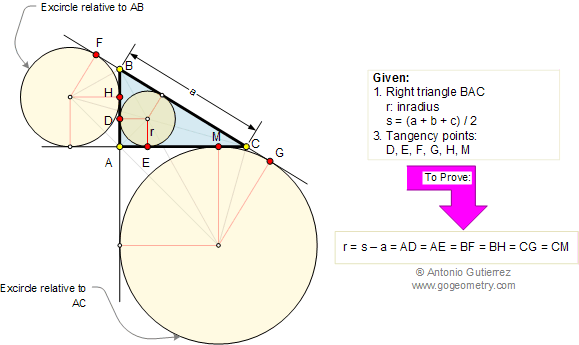Problem 200. Right Triangle, Incircle, Excircles, Points of Tangency, Inradius
 In the figure below, BAC is a right triangle with the incircle of radius r and the excircles relatives to the legs or catheti (singular: cathetus). If s is the semiperimeter and D, E, F, G, H, and M are the points of tangency, prove that r = s - a = AD = AE = BF = BH = CG = CM. View or post a solution.Home | Geometry | Search | Problems | 191-200 | Email